## 56) Partitioning Subtraction

Ten Plus Bonds: Strategy Five Plus Bonds### Mathematics

Develop the strategy of partitioning a whole up to 20, into two parts, using five plus bonds, to solve subtraction.

For example, 12 – 5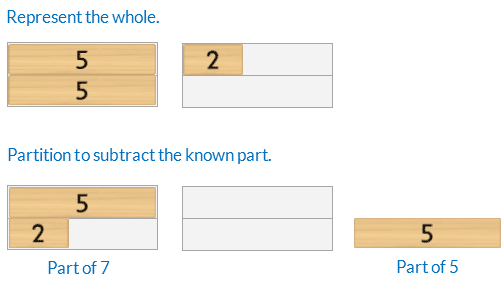For example, 18 – 5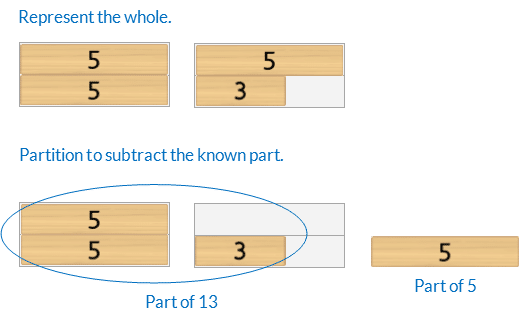Prior knowledge of the five plus bonds, commutative property of addition, part-part-whole and place value partitioning is needed.

### Language

• subtract
• equals
• five plus bond
• partitioning
• part-part-whole

Note, in this activity students are finding a missing part in a subtraction equation.

They are NOT completing “sums”. The “sum” is the whole, found by adding the parts.

The word ‘sum’ is often incorrectly used when the word ‘equation’ is meant. It is impossible to “complete a subtraction sum”.## Differentiation

### A little easier

##### Five plus bond revision

Play Five Plus Bonds: Subtraction Building A Wall to develop fluency verbalising the wholes of 6,7,8,9 as five plus bond subtraction equations.##### Counting order

Calculate each question in counting order, working clockwise around the spinner, from the 12 o’clock position. Do not flick the spinner. Assist students to identify patterns.

### A little harder

##### Partitioning subtraction strategy five plus bonds

Play Ten Plus Bonds: Partitioning Subtraction Strategy Five Plus Bonds a little harder to develop the strategy of partitioning wholes up to 30, into two parts, using five plus bonds, to solve subtraction.

For example, 22 – 5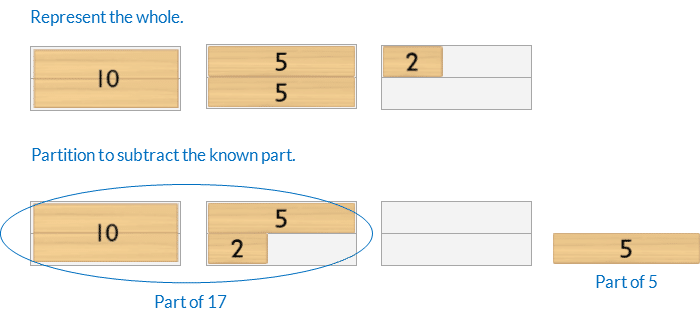For example, 28 – 5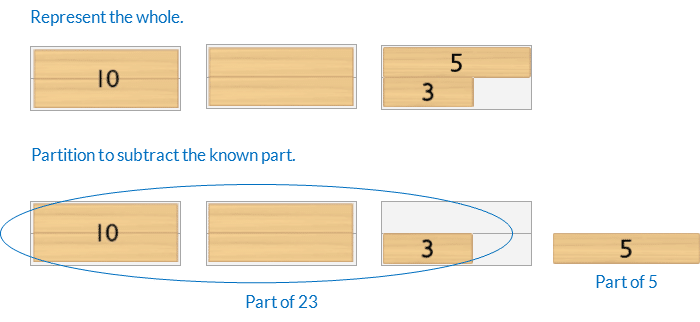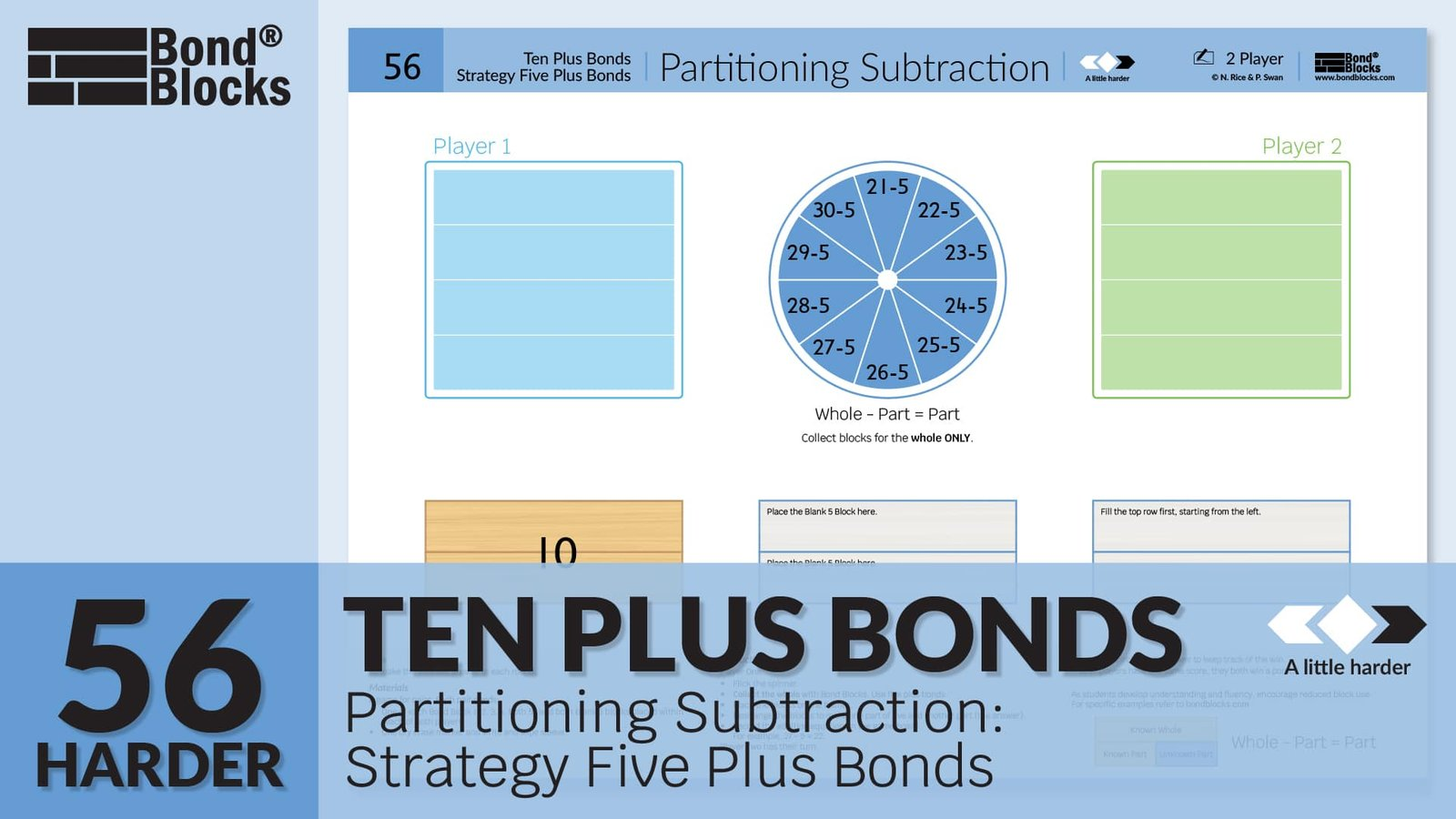##### Reduce scaffolds

The blocks are a scaffold to support calculation. If students can calculate without some or all of the blocks encourage this. Below is a progression of reducing scaffolding, from most to least support, using partitioning with five plus bonds for 22 – 5. Subtraction is solved by thinking about part-part-whole where the whole is partitioned using five plus bonds.### Progression

This chapter of activities has focused on two-digit wholes from 10 to 20 partitioned using ten plus bonds (place value). The next chapter of activities focuses on the same wholes but partitions them with double bonds. Go to

##### Activity 57

Doubling and Halving to 20: Bonds, Building a Wall